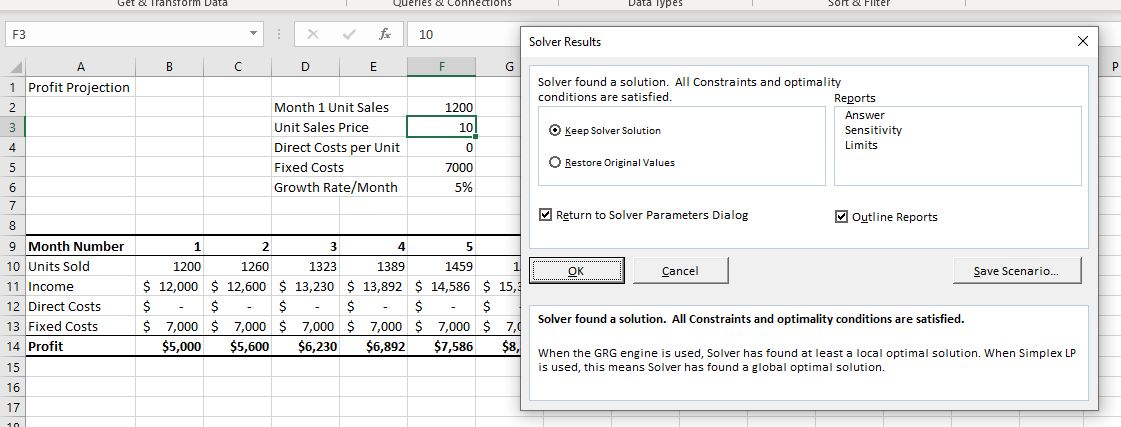# How to Fix When Excel Solver Encountered an Error Value

This tutorial demonstrates how to fix the issue when “Solver encountered an error value.”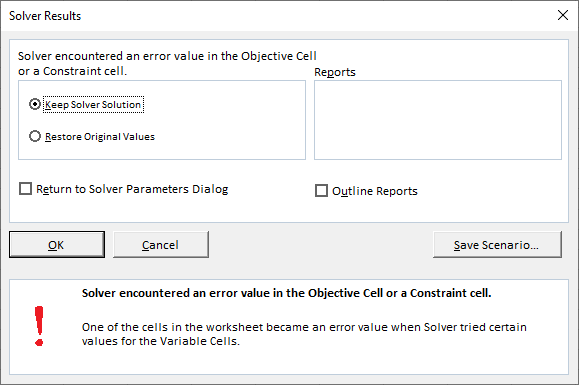Solver is an add-in that is provided with Excel and is used to perform “what-if” analyses by providing alternative answers to a formula in a cell based on values you may pass to the formula from other cells in your workbook.

## Worksheet Formula Error

One reason for an error in Solver occurring could be a worksheet formula error. It may be that, as Solver performs the operation, it finds an error in a formula on the worksheet and therefore cannot continue.

For example, consider the example below: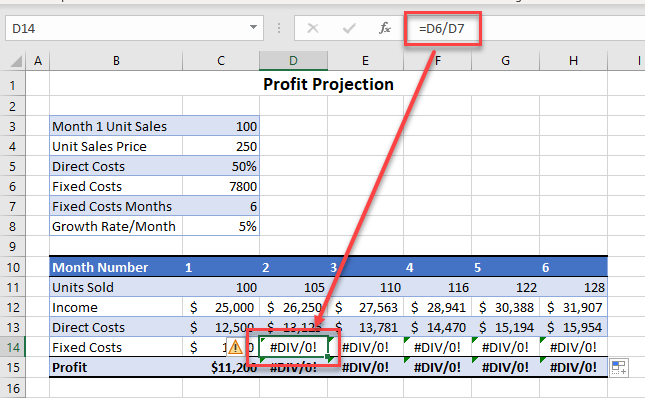If you wish to use Solver, you first need to make sure you don’t have any errors in your worksheet that cause Solver to fail. This worksheet has a number of #DIV/0 errors. If you attempt to use Solver on any of these cells, you get the error Solver encountered an error value in the Objective Cell or a Constraint Cell.

Amend the formula to correct it and you can then continue to use Solver.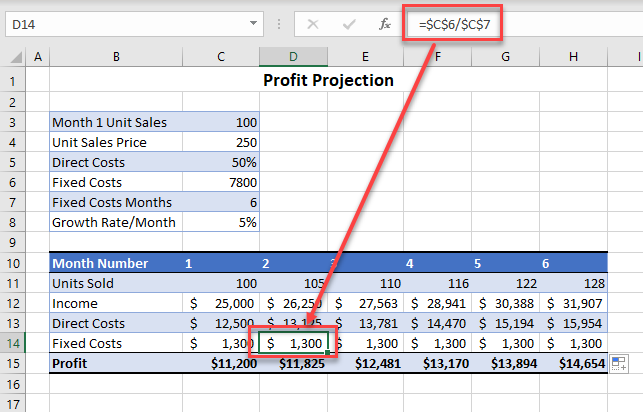To make sure there are not any errors in your worksheet prior to using Solver, you can use the Error Checking command in Excel.

## Solver Formula Error

Solver itself might cause a formula in your worksheet to end up returning an error value. If this is the case, the error One of the cells in the worksheet became an error value when Solver tried certain values for the Variable Cells is returned and Solver gets to the point where it cannot continue as, when it continues, it is encountering error values.

To fix this problem, you would need to amend the constraints. As the error has occurred in one of the constraint formulas, it is difficult to find the error on the screen as the normal #DIV/0 that one would get when a formula tries to divide by zero does not occur.

For example, consider the constraints set below: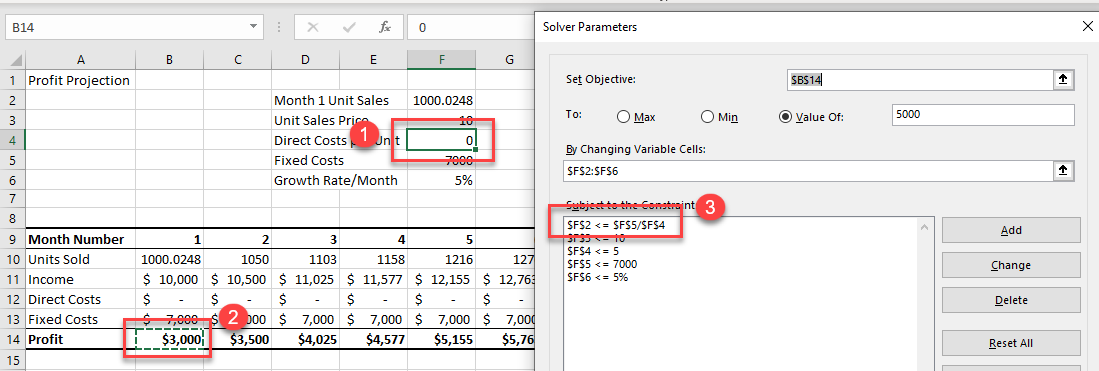Rather than using formulas in the constraints, use constant numbers or cells containing constant numbers such as the amended constraints below. You have removed the formula from the first constraint and replaced it with a number.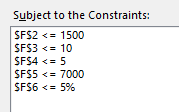Now when you try to solve, you are successful.